# Problem 57760. Easy Sequences 101: One-line Code Challenge - n-th Digit of Fibonacci Sequence

For a given index x, the x-th Fibonacci number,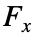is defined as: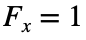for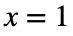or 2, and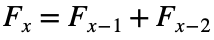for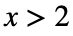.
What this problem requires is find the digit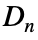, which is the n-th digit when the Fibonacci numbers are laid side by side.
For example, for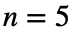,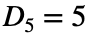, and if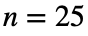,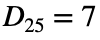.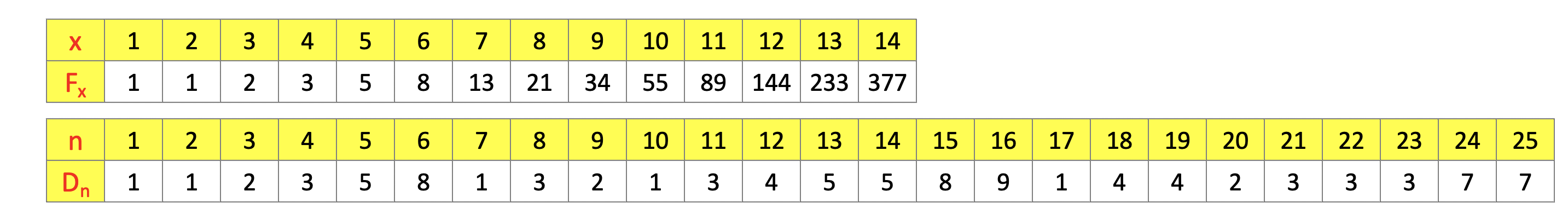--------------------------------
NOTE: The following restrictions apply:
• The function should only have one (1) line of code, excluding the function start line.
• Semicolons (;) are considered end-of-line characters.
• Please suppress the function end line. Keyword 'end' is not allowed.
• Importing libraries is not allowed.
• Regular expressions and string manipulation are not allowed.

### Solution Stats

0.0% Correct | 100.0% Incorrect
Last Solution submitted on Oct 19, 2023

#### Problem Recent Solvers0

No solvers yet, be the first player to solve this problem.

### Community Treasure Hunt

Find the treasures in MATLAB Central and discover how the community can help you!

Start Hunting!